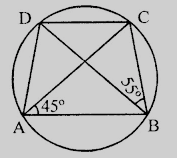"
">

# In the figure, $ABCD$ is a cyclic quadrilateral in which $AC$ and $BD$ are its diagonals. If $\angle DBC = 55^o$ and $\angle BAC = 45^o$, find $\angle BCD$."

Given:

$ABCD$ is a cyclic quadrilateral in which $AC$ and $BD$ are its diagonals.

$\angle DBC = 55^o$ and $\angle BAC = 45^o$.

To do:

We have to find $\angle BCD$.

Solution:

$\angle BAC$ and $\angle BDC$ are in the same segment.

This implies,

$\angle BAC = \angle BDC = 45^o$

In $\triangle BCD$,

$\angle DBC + \angle BDC + \angle BCD = 180^o$       (Sum of angles of a triangle)

$55^o + 45^o + \angle BCD = 180^o$

$100^o + \angle BCD = 180^o$

$\angle BCD = 180^o - 100^o = 80^o$

Hence $\angle BCD = 80^o$.

Updated on: 10-Oct-2022

33 Views